Half-Life and Activity

# Half-Life and Activity - Notes | Study Modern Physics for IIT JAM - Physics

 1 Crore+ students have signed up on EduRev. Have you?

Half-Life
Why use a term like half-life rather than lifetime? The answer can be found by examining , which shows how the number of radioactive nuclei in a sample decreases with time. The time in which half of the original number of nuclei decay is defined as the half-life, t1/2. Half of the remaining nuclei decay in the next half-life. Further, half of that amount decays in the following half-life. Therefore, the number of radioactive nuclei decreases from N to N/2 in one half-life, then to N/4 in the next, and to N/8 in the next, and so on. If N is a large number, then many half-lives (not just two) pass before all of the nuclei decay. Nuclear decay is an example of a purely statistical process. A more precise definition of half-life is that each nucleus has a 50% chance of living for a time equal to one half-life t1/2. Thus, if N is reasonably large, half of the original nuclei decay in a time of one half-life. If an individual nucleus makes it through that time, it still has a 50% chance of surviving through another half-life. Even if it happens to make it through hundreds of half-lives, it still has a 50% chance of surviving through one more. The probability of decay is the same no matter when you start counting. This is like random coin flipping. The chance of heads is 50%, no matter what has happened before.
Radioactive decay reduces the number of radioactive nuclei over time. In one half-life t1/2, the number decreases to half of its original value. Half of what remains decay in the next half-life, and half of those in the next, and so on. This is an exponential decay, as seen in the graph of the number of nuclei present as a function of time.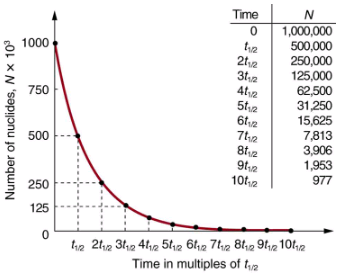There is a tremendous range in the half-lives of various nuclides, from as short as 10-23 s for the most unstable, to more than 1016 y for the least unstable, or about 46 orders of magnitude. Nuclides with the shortest half-lives are those for which the nuclear forces are least attractive, an indication of the extent to which the nuclear force can depend on the particular combination of neutrons and protons. The concept of half-life is applicable to other subatomic particles, as will be discussed in Particle Physics. It is also applicable to the decay of excited states in atoms and nuclei. The following equation gives the quantitative relationship between the original number of nuclei present at time zero (N0) and the number (N) at a later time t:
N = N0e-λt,
where e = 2.71828... is the base of the natural logarithm, and λ is the decay constant for the nuclide. The shorter the half-life, the larger is the value of λ, and the faster the exponential e-λt decreases with time. The relationship between the decay constant λ and the half-life t1/2 isTo see how the number of nuclei declines to half its original value in one half-life, let t= t1/2 in the exponential in the equation N = N0e-λt. This gives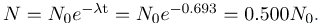For integral numbers of half-lives, you can just divide the original number by 2 over and over again, rather than using the exponential relationship. For example, if ten half-lives have passed, we divide N by 2 ten times. This reduces it to N/1024. For an arbitrary time, not just a multiple of the half-life, the exponential relationship must be used.
Radioactive dating is a clever use of naturally occurring radioactivity. Its most famous application is carbon-14 dating. Carbon-14 has a half-life of 5730 years and is produced in a nuclear reaction induced when solar neutrinos strike 14N in the atmosphere. Radioactive carbon has the same chemistry as stable carbon, and so it mixes into the ecosphere, where it is consumed and becomes part of every living organism. Carbon-14 has an abundance of 1.3 parts per trillion of normal carbon. Thus, if you know the number of carbon nuclei in an object (perhaps determined by mass and Avogadro’s number), you multiply that number by 1.310-12 to find the number of
14C nuclei in the object. When an organism dies, carbon exchange with the environment ceases, and
14C is not replenished as it decays. By comparing the abundance of
14C in an artifact, such as mummy wrappings, with the normal abundance in living tissue, it is possible to determine the artifact’s age (or time since death). Carbon-14 dating can be used for biological tissues as old as 50 or 60 thousand years, but is most accurate for younger samples, since the abundance of
14C nuclei in them is greater. Very old biological materials contain no
14C at all. There are instances in which the date of an artifact can be determined by other means, such as historical knowledge or tree-ring counting. These cross-references have confirmed the validity of carbon-14 dating and permitted us to calibrate the technique as well. Carbon-14 dating revolutionized parts of archaeology and is of such importance that it earned the 1960 Nobel Prize in chemistry for its developer, the American chemist Willard Libby (1908–1980).
One of the most famous cases of carbon-14 dating involves the Shroud of Turin, a long piece of fabric purported to be the burial shroud of Jesus. This relic was first displayed in Turin in 1354 and was denounced as a fraud at that time by a French bishop. Its remarkable negative imprint of an apparently crucified body resembles the then-accepted image of Jesus, and so the shroud was never disregarded completely and remained controversial over the centuries. Carbon-14 dating was not performed on the shroud until 1988, when the process had been refined to the point where only a small amount of material needed to be destroyed. Samples were tested at three independent laboratories, each being given four pieces of cloth, with only one unidentified piece from the shroud, to avoid prejudice. All three laboratories found samples of the shroud contain 92% of the 14C found in living tissues, allowing the shroud to be dated.
Part of the Shroud of Turin, which shows a remarkable negative imprint likeness of Jesus complete with evidence of crucifixion wounds. The shroud first surfaced in the 14th century and was only recently carbon-14 dated. It has not been determined how the image was placed on the material. (credit: Butko, Wikimedia Commons)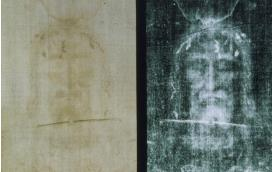How Old Is the Shroud of Turin?
Calculate the age of the Shroud of Turin given that the amount of 14C found in it is 92% of that in living tissue.
Strategy
Knowing that 92% of the 14C remains means that N/N0 = 0.92. Therefore, the equation N = N0e-λt can be used to find λt. We also know that the half-life of 14C is 5730 y, and so once λt is known, we can use the equation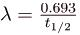to find λ and then find t as requested. Here, we postulate that the decrease in 14C is solely due to nuclear decay.
Solution
Solving the equation N = N0e-λt for N/Ngives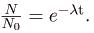Thus,
0.92 = e-λt .
Taking the natural logarithm of both sides of the equation yields
In 0.92 = -λt
so that
-0.0834 = -λt
Rearranging to isolate t gives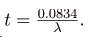Now, the equation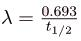can be used to find λ for 14C. Solving for λ and substituting the known half-life gives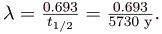We enter this value into the previous equation to find t: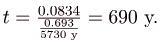Discussion
This dates the material in the shroud to 1988–690 = a.d. 1300. Our calculation is only accurate to two digits, so that the year is rounded to 1300. The values obtained at the three independent laboratories gave a weighted average date of a.d. 132060. The uncertainty is typical of carbon-14 dating and is due to the small amount of 14C in living tissues, the amount of material available, and experimental uncertainties (reduced by having three independent measurements). It is meaningful that the date of the shroud is consistent with the first record of its existence and inconsistent with the period in which Jesus lived.
There are other forms of radioactive dating. Rocks, for example, can sometimes be dated based on the decay of  238U. The decay series for 238U ends with 206Pb, so that the ratio of these nuclides in a rock is an indication of how long it has been since the rock solidified. The original composition of the rock, such as the absence of lead, must be known with some confidence. However, as with carbon-14 dating, the technique can be verified by a consistent body of knowledge. Since 238U has a half-life of 4.5109 y, it is useful for dating only very old materials, showing, for example, that the oldest rocks on Earth solidified about 3.5109 years ago.
Activity, the Rate of Decay
What do we mean when we say a source is highly radioactive? Generally, this means the number of decays per unit time is very high. We define activity R to be the rate of decay expressed in decays per unit time. In equation form, this is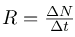where N is the number of decays that occur in time t. The SI unit for activity is one decay per second and is given the name becquerel (Bq) in honor of the discoverer of radioactivity. That is,
1 Bq = 1 dacay/s.
Activity R is often expressed in other units, such as decays per minute or decays per year. One of the most common units for activity is the curie (Ci), defined to be the activity of 1 g of 226Ra, in honor of Marie Curie’s work with radium. The definition of curie is
1 Ci = 3.701010 Bq,
or 3.701010 decays per second. A curie is a large unit of activity, while a becquerel is a relatively small unit. 1 MBq = 100 microcuries (μCi). In countries like Australia and New Zealand that adhere more to SI units, most radioactive sources, such as those used in medical diagnostics or in physics laboratories, are labeled in Bq or megabecquerel (MBq).
Intuitively, you would expect the activity of a source to depend on two things: the amount of the radioactive substance present, and its half-life. The greater the number of radioactive nuclei present in the sample, the more will decay per unit of time. The shorter the half-life, the more decays per unit time, for a given number of nuclei. So activity R should be proportional to the number of radioactive nuclei, N, and inversely proportional to their half-life, t1/2. In fact, your intuition is correct. It can be shown that the activity of a source is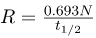where N is the number of radioactive nuclei present, having half-life  t1/2. This relationship is useful in a variety of calculations, as the next two examples illustrate.
How Great Is the 14C Activity in Living Tissue?
Calculate the activity due to 14C in 1.00 kg of carbon found in a living organism. Express the activity in units of Bq and Ci.
Strategy
To find the activity R using the equation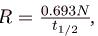we must know N and t1/2. The half-life of 14C can be found in Appendix B, and was stated above as 5730 y. To find N, we first find the number of 12C nuclei in 1.00 kg of carbon using the concept of a mole. As indicated, we then multiply by 1.310-12 (the abundance of 14C in a carbon sample from a living organism) to get the number of 14C nuclei in a living organism.
Solution
One mole of carbon has a mass of 12.0 g, since it is nearly pure 12C. (A mole has a mass in grams equal in magnitude to A found in the periodic table.) Thus the number of carbon nuclei in a kilogram is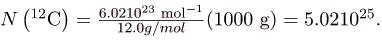So the number of 14C nuclei in 1 kg of carbon is
N(14C) = (5.021025) (1.31012) = 6.521013.
Now the activity R is found using the equation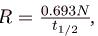Entering known values gives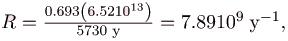or 7.89109 decays per year. To convert this to the unit Bq, we simply convert years to seconds. Thus,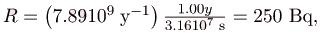or 250 decays per second. To express R in curies, we use the definition of a curie,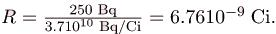Thus, R = 6.76 nCi.
Discussion
Our own bodies contain kilograms of carbon, and it is intriguing to think there are hundreds of 14C decays per second taking place in us. Carbon-14 and other naturally occurring radioactive substances in our bodies contribute to the background radiation we receive. The small number of decays per second found for a kilogram of carbon in this example gives you some idea of how difficult it is to detect 14C in a small sample of material. If there are 250 decays per second in a kilogram, then there are 0.25 decays per second in a gram of carbon in living tissue. To observe this, you must be able to distinguish decays from other forms of radiation, in order to reduce background noise. This becomes more difficult with an old tissue sample, since it contains less 14C, and for samples more than 50 thousand years old, it is impossible.
Human-made (or artificial) radioactivity has been produced for decades and has many uses. Some of these include medical therapy for cancer, medical imaging and diagnostics, and food preservation by irradiation. Many applications as well as the biological effects of radiation are explored in Medical Applications of Nuclear Physics, but it is clear that radiation is hazardous. A number of tragic examples of this exist, one of the most disastrous being the meltdown and fire at the Chernobyl reactor complex in the Ukraine. Several radioactive isotopes were released in huge quantities, contaminating many thousands of square kilometers and directly affecting hundreds of thousands of people. The most significant releases were of 131I, 90Sr, 137Cs, 239Pu, 238U, and 235U.
Estimates are that the total amount of radiations released was about 100 million curies.
Human and Medical Applications
The Chernobyl reactor. More than 100 people died soon after its meltdown, and there will be thousands of deaths from radiation-induced cancer in the future. While the accident was due to a series of human errors, the cleanup efforts were heroic. Most of the immediate fatalities were firefighters and reactor personnel.What Mass of 137Cs Escaped Chernobyl?
It is estimated that the Chernobyl disaster released 6.0 MCi of 137Cs into the environment. Calculate the mass of 137Cs released.
Strategy
We can calculate the mass released using Avogadro’s number and the concept of a mole if we can first find the number of nuclei N released. Since the activity R is given, and the half-life of 137Cs is found in Appendix B to be 30.2 y, we can use the equation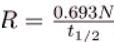to find N.
Solution

Solving the equation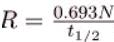for N gives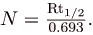Entering the given values yields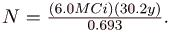Converting curies to becquerels and years to seconds, we get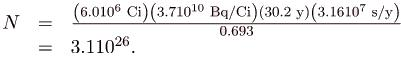One mole of a nuclide AX has a mass of A grams, so that one mole of 137Cs has a mass of 137 g. A mole has 6.021023 nuclei. Thus the mass of 137Cs released was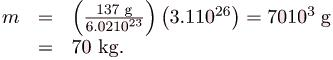Discussion
While 70 kg of material may not be a very large mass compared to the amount of fuel in a power plant, it is extremely radioactive, since it only has a 30-year half-life. Six megacuries (6.0 MCi) is an extraordinary amount of activity but is only a fraction of what is produced in nuclear reactors. Similar amounts of the other isotopes were also released at Chernobyl. Although the chances of such a disaster may have seemed small, the consequences were extremely severe, requiring greater caution than was used. More will be said about safe reactor design in the next chapter, but it should be noted that Western reactors have a fundamentally safer design.
Activity R decreases in time, going to half its original value in one half-life, then to one-fourth its original value in the next half-life, and so on. Since, the activity decreases as the number of radioactive nuclei decreases. The equation for R as a function of time is found by combining the equations N = N0e-λt andyielding
R = Roe-λt,
where R0 is the activity at t = 0. This equation shows exponential decay of radioactive nuclei. For example, if a source originally has a 1.00-mCi activity, it declines to 0.500 mCi in one half-life, to 0.250 mCi in two half-lives, to 0.125 mCi in three half-lives, and so on. For times other than whole half-lives, the equation R = Roe-λt, must be used to find R.
Section Summary

• Half-life t1/2 is the time in which there is a 50% chance that a nucleus will decay. The number of nuclei N as a function of time is
N = N0e-λt,
where N0 is the number present at t=0, and λ is the decay constant, related to the half-life by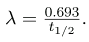• One of the applications of radioactive decay is radioactive dating, in which the age of a material is determined by the amount of radioactive decay that occurs. The rate of decay is called the activity R: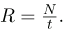• The SI unit for R is the becquerel (Bq), defined by
1 Bq = 1 decay/s.
• R is also expressed in terms of curies (Ci), where
1 Ci = 3.701010 Bq.
• The activity R of a source is related to N and  t1/2 by• Since N has an exponential behavior as in the equation N = N0e-λt, the activity also has an exponential behavior, given by
R = Roe-λt,
where R0 is the activity at t=0.

Conceptual Questions
In a 3109 -year-old rock that originally contained some
2358U, which has a half-life of
4.5109 years, we expect to find some years, we expect to find some 2358U remaining in it. Why are 226Ra, 222Rn, and 210Po also found in such a rock, even though they have much shorter half-lives (1600 years, 3.8 days, and 138 days, respectively)?
Does the number of radioactive nuclei in a sample decrease to exactly half its original value in one half-life? Explain in terms of the statistical nature of radioactive decay.
Radioactivity depends on the nucleus and not the atom or its chemical state. Why, then, is one kilogram of uranium more radioactive than one kilogram of uranium hexafluoride?
Explain how a bound system can have less mass than its components. Why is this not observed classically, say for a building made of bricks?
Spontaneous radioactive decay occurs only when the decay products have less mass than the parent, and it tends to produce a daughter that is more stable than the parent. Explain how this is related to the fact that more tightly bound nuclei are more stable. (Consider the binding energy per nucleon.)
To obtain the most precise value of BE from the equation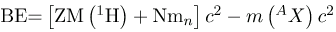, we should take into account the binding energy of the electrons in the neutral atoms. Will doing this produce a larger or smaller value for BE? Why is this effect usually negligible?
How does the finite range of the nuclear force relate to the fact that BE/A is greatest for A near 60?
Problems & Exercises
Data from the appendices and the periodic table may be needed for these problems.An old campfire is uncovered during an archaeological dig. Its charcoal is found to contain less than 1/1000 the normal amount of 14C. Estimate the minimum age of the charcoal, noting that 210=1024.
57,300 yA 60Co source is labeled 4.00 mCi, but its present activity is found to be 1.85107 Bq.
(a) What is the present activity in mCi?
(b) How long ago did it actually have a 4.00-mCi activity?
(a) Calculate the activity R in curies of 1.00 g of 226Ra. (b) Discuss why your answer is not exactly 1.00 Ci, given that the curie was originally supposed to be exactly the activity of a gram of radium.
(a) 0.988 Ci
(b) The half-life of 226Ra is now better known.
Show that the activity of the 14C in 1.00 g of 12C found in living tissue is 0.250 Bq.
Mantles for gas lanterns contain thorium, because it forms an oxide that can survive being heated to incandescence for long periods of time. Natural thorium is almost 100% 232Th, with a half-life of 1.4051010 y. If an average lantern mantle contains 300 mg of thorium, what is its activity?
1.22103 Bq
Cow’s milk produced near nuclear reactors can be tested for as little as 1.00 pCi of 131I per liter, to check for possible reactor leakage. What mass of 131I has this activity?
(a) Natural potassium contains 40K, which has a half-life of 1.277109 y. What mass of 40K in a person would have a decay rate of 4140 Bq?
(b) What is the fraction of 40K in natural potassium, given that the person has 140 g in his body? (These numbers are typical for a 70-kg adult.
(a) 16.0 mg
(b) 0.0114%
There is more than one isotope of natural uranium. If a researcher isolates 1.00 mg of the relatively scarce 235U and finds this mass to have an activity of 80.0 Bq, what is its half-life in years?
50V has one of the longest known radioactive half-lives. In a difficult experiment, a researcher found that the activity of 1.00 kg of 50V is 1.75 Bq. What is the half-life in years
1.481017 y
You can sometimes find deep red crystal vases in antique stores, called uranium glass because their color was produced by doping the glass with uranium. Look up the natural isotopes of uranium and their half-lives, and calculate the activity of such a vase assuming it has 2.00 g of uranium in it. Neglect the activity of any daughter nuclides.
A tree falls in a forest. How many years must pass before the 14C activity in 1.00 g of the tree’s carbon drops to 1.00 decay per hour?
5.6104 y
What fraction of the 40K that was on Earth when it formed 4.5109 years ago is left today?
A 5000-Ci 60Co source used for cancer therapy is considered too weak to be useful when its activity falls to 3500 Ci. How long after its manufacture does this happen?
2.71 y
Natural uranium is 0.7200% 235U and 99.27% 238U. What were the percentages of 235U and 238U in natural uranium when Earth formed 4.5109 years ago?
The β- particles emitted in the decay of 3H (tritium) interact with matter to create light in a glow-in-the-dark exit sign. At the time of manufacture, such a sign contains 15.0 Ci of 3H.
(a) What is the mass of the tritium?
(b) What is its activity 5.00 y after manufacture?
(a) 1.56 mg
(b) 11.3 Ci
World War II aircraft had instruments with glowing radium-painted dials. The activity of one such instrument was 1.0105 Bq when new.
(a) What mass of 226Ra was present?
(b) After some years, the phosphors on the dials deteriorated chemically, but the radium did not escape. What is the activity of this instrument 57.0 years after it was made?
(a) The 210Po source used in a physics laboratory is labeled as having an activity of 1.0 μCi on the date it was prepared. A student measures the radioactivity of this source with a Geiger counter and observes 1500 counts per minute. She notices that the source was prepared 120 days before her lab. What fraction of the decays is she observing with her apparatus?
(b) Identify some of the reasons that only a fraction of the α s emitted are observed by the detector.
(a) 1.2310-3
(b) Only part of the emitted radiation goes in the direction of the detector. Only a fraction of that causes a response in the detector. Some of the emitted radiation (mostly α particles) is observed within the source. Some is absorbed within the source, some is absorbed by the detector, and some does not penetrate the detector.Armor-piercing shells with depleted uranium cores are fired by aircraft at tanks. (The high density of the uranium makes them effective.) The uranium is called depleted because it has had its 235U removed for reactor use and is nearly pure 238U . Depleted uranium has been erroneously called non-radioactive. To demonstrate that this is wrong:
(a) Calculate the activity of 60.0 g of pure 238U.
(b) Calculate the activity of 60.0 g of natural uranium, neglecting the 234U and all daughter nuclides.
The ceramic glaze on a red-orange Fiestaware plate is U2O3 and contains 50.0 grams of 238U, but very little 238U.
(a) What is the activity of the plate?
(b) Calculate the total energy that will be released by 238U decay.
(c) If energy is worth 12.0 cents per k.h, what is the monetary value of the energy emitted? (These plates went out of production some 30 years ago, but are still available as collectibles.)
(a) 1.6810-5 Ci
(b) 8.651010 J
(c) 2.9103
Large amounts of depleted uranium (238U) are available as a by-product of uranium processing for reactor fuel and weapons. Uranium is very dense and makes good counter weights for aircraft. Suppose you have a 4000-kg block of 238U.
(a) Find its activity.
(b) How many calories per day are generated by thermalization of the decay energy?
(c) Do you think you could detect this as heat? Explain.The Galileo space probe was launched on its long journey past several planets in 1989, with an ultimate goal of Jupiter. Its power source is 11.0 kg of 238Pu, a by-product of nuclear weapons plutonium production. Electrical energy is generated thermoelectrically from the heat produced when the 5.59-MeV
particles emitted in each decay crash to a halt inside the plutonium and its shielding. The half-life of 238Pu is 87.7 years.
(a) What was the original activity of the 238Pu in becquerel?
(b) What power was emitted in kilowatts?
(c) What power was emitted 12.0 y after launch? You may neglect any extra energy from daughter nuclides and any losses from escaping γ rays.
(a) 6.971015 Bq
(b) 6.24 kW
(c) 5.67 kW
Consider the generation of electricity by a radioactive isotope in a space probe, such as described in. Construct a problem in which you calculate the mass of a radioactive isotope you need in order to supply power for a long space flight. Among the things to consider are the isotope chosen, its half-life and decay energy, the power needs of the probe and the length of the flight.
Unreasonable Results
A nuclear physicist finds
1.0 μg of 236U in a piece of uranium ore and assumes it is primordial since its half-life is
2.3107 y.
(a) Calculate the amount of 236U that would had to have been on Earth when it formed
4.5109 y ago for 1.0 μg to be left today.
(c) What assumption is responsible?
Unreasonable Results
(a) Repeat but include the 0.0055% natural abundance of 234U
with its
2.45105 y half-life.
(c) What assumption is responsible?
(d) Where does the 234U come from if it is not primordial?
Unreasonable Results
The manufacturer of a smoke alarm decides that the smallest current of radiation he can detect is
1.0 μA.
(a) Find the activity in curies of an emitter that produces a 1.0 μA current of particles.
(c) What assumption is responsible?
(a) 84.5 Ci
(b) An extremely large activity, many orders of magnitude greater than permitted for home use.
(c) The assumption of 1.00 A is unreasonably large. Other methods can detect much smaller decay rates.

The document Half-Life and Activity - Notes | Study Modern Physics for IIT JAM - Physics is a part of the Physics Course Modern Physics for IIT JAM.
All you need of Physics at this link: Physics

## Modern Physics for IIT JAM

54 videos|44 docs|15 tests
 Use Code STAYHOME200 and get INR 200 additional OFF

## Modern Physics for IIT JAM

54 videos|44 docs|15 tests

Track your progress, build streaks, highlight & save important lessons and more!

,

,

,

,

,

,

,

,

,

,

,

,

,

,

,

,

,

,

,

,

,

;